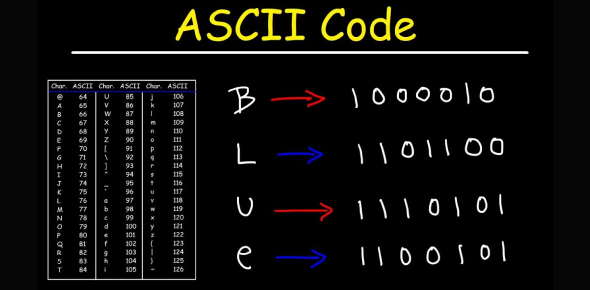# Convert ASCII To Binary Quiz! Test

Approved & Edited by ProProfs Editorial Team
At ProProfs Quizzes, our dedicated in-house team of experts takes pride in their work. With a sharp eye for detail, they meticulously review each quiz. This ensures that every quiz, taken by over 100 million users, meets our standards of accuracy, clarity, and engagement.
| Written by Mazesarecool
M
Mazesarecool
Community Contributor
Quizzes Created: 1 | Total Attempts: 947
Questions: 26 | Attempts: 947Settings.

• 1.

• 2.

• 3.

### C

Explanation
The given answer is the same as the input, which suggests that the input is a binary number. The binary number system uses only two digits, 0 and 1, to represent numbers. In this case, the binary number 1100011 represents the decimal number 99.

Rate this question:

• 4.

• 5.

• 6.

• 7.

• 8.

• 9.

### I

Explanation
The given answer is the same as the given input, which is "1101001". Therefore, the answer is correct as it matches the input exactly.

Rate this question:

• 10.

• 11.

• 12.

### L

Explanation
The given answer is the same as the given input, which is 1101100. This indicates that the answer is simply a repetition or duplication of the input.

Rate this question:

• 13.

• 14.

• 15.

### O

Explanation
The given answer is the same as the given sequence of numbers.

Rate this question:

• 16.

• 17.

### Q

Explanation
The given answer is the same as the question, "1110001". Since there is no additional information or context provided, it can be assumed that the answer is simply a repetition of the given input.

Rate this question:

• 18.

### R

Explanation
The given answer is the same as the given number "1110010".

Rate this question:

• 19.

• 20.

• 21.

### U

Explanation
The given answer is the same as the given input. Therefore, it is correct.

Rate this question:

• 22.

• 23.

### W

Explanation
The given answer is the same as the given input sequence "1110111". Therefore, it is the correct answer.

Rate this question:

• 24.

• 25.

• 26.

### Z

Related TopicsBack to top
×

Wait!
Here's an interesting quiz for you.